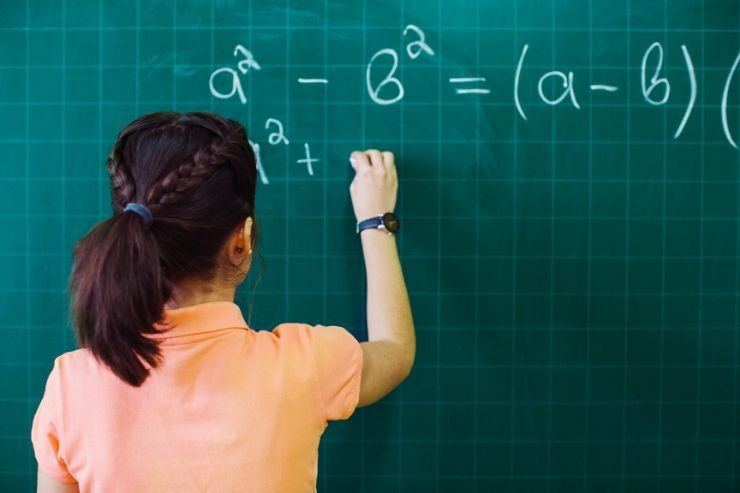# Tips to attempt ICSE Class 10 Board Exam – MathsMathematics tops the list of subjects as the ‘highest scoring subject of all’ in a board exam. Scoring high in mathematics not only improves your aggregate, but also takes you a step closer to your preferred career choices – engineering, data analytics, business statistics etc!

Over the years, teachers have emphasized on the significance of regular practice across the year as the key to scoring high in the final board exam.  Ajay Singh- ICSE Board Examiner and a Teacher at Ryan International School, Malad ICSE shares key insights for students to keep in mind while attempting Class 10 ICSE Board Exam Maths paper.

Let’s cover the basis first!

• Some of the key subjects in the ICSE Class 10 Maths Paper are Probability, Statistics, Algebra, Geometry, Trigonometry, Commercial Mathematics and Mensuration
• The ICSE Class 10 Maths board exams comprises of 1 external exams for 80 marks and 1 internal assessment for 20 marks.
• External exams last for 2.5 hours wherein the paper is divided in 2 parts. Section-A – where in the students answer 4 questions compulsorily for a total of 40 marks and Section-B where students answer 4 questions out of 7 questions for a total 40 marks

Moving on, once at the exam hall, here are some tips for students should attempt the maths paper to score high marks

## Tips To Solve ICSE Class 10 Maths Exam Paper:

1. Reading Time: 15 Minutes (Not Included in 2.5 hours)

• Start reading the questions in Section-A
• After Section A , refer to Section-B and choose the 4 questions you wish to attempt. I suggest deciding on one extra question.
• By end of reading time of 15 minutes, you will have most of the questions solved mentally
• I suggest students read the question paper at-least 2 times

2. Writing Time: 135 Minutes

• Start answer questions from Section-A onward. Don’t waste time and be quick
• Strictly do not change the order questions in Section-A
• Spend approximately 60 minutes for solving Section-A. Do not leave any question unattended
• Spend approximately 60 minutes for solving Section-B
• Use the remaining 15 minutes to compete the pending questions from any of the sections
• You can change the order of solving the questions in Section-B
• Here are some subject matter tips
• Commercial Arithmetic/Algebra: Read the question carefully and meticulously consider all the given facts/data while solving
• Word Problems(GST, Solving Problems-Quadratic Equations, Similarity, Cylinder-Cone-Sphere, Heights & distances, Probability etc) : Read the questions at-least 2 times and try to figure out the correlation of data provided in the questions. Put down all the assumptions made by you clearly on the paper
• Geometry/Coordinates Geometry: Draw the figure whenever possible so that you have an understanding about the steps needed for complete and correct solution
• Constructions: Steps of construction are not required, but a rough figure is always better to have an idea of tricks/steps needed for correct construction
• Graph: Don’t forget to mention the scale in the graph. Use a dark pencil(do not use pen) for ogive and other types of graphs related questions an Write the formula whenever possible for all answers.
• Missing essential steps while writing your mathematics paper will result in deduction of marks. So remember to write down all the steps without actually missing anything.

3. Review Time: 15 Minutes

• Last 15 minutes, only review/ revise the answers written.
• Begin the reviewing from Section-A followed by Section-B.
• Review Section-A and Section-B at least 2 times.